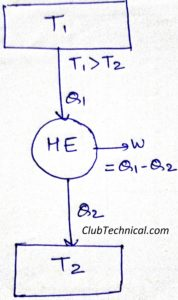# Clausius’ Inequality and Clausius’ Theorem

By | March 5, 2019

## Clausius’ theorem

The cyclic integral of ∂Q/T for a reversible cycle is always equal to zero.

Proof of Clausius’ theorem:

Let’s consider a reversible heat engine.From the absolute thermodynamic scale of temperature, we know that for a reversible process

Q1/Q2 = T1/T2

Q1/T1 – Q2/T2 = 0

Q1/T1 + (-Q2)/T2 = 0

ΣCYCLE (Q/T) = 0

In terms of cyclic integral we can write above equation as

CYCLE (∂QR/T) = 0   … (1)

Hence proved that the cyclic integral of ∂Q/T for a reversible cycle is always equal to zero.

## Clausius’ inequality

The cyclic integral of ∂Q/T for an irreversible process is always less than ∂Q/T for a reversible process (both operating between the same temperature limits).

Proof of Clausius’ inequality:

Let’s consider two heat engines one is reversible and another is irreversible, operating between the same temperature limits.Efficiency of reversible engine

η = 1 – (Q2/Q1)

Efficiency of irreversible engine

ηR = 1 – (Q2R/Q1R)

From the Carnot’s theorem we know that

Efficiency of a reversible heat engine is always more than the efficiency of an irreversible heat engine (operating between the same temperature limits).

ηR > η

1 – (Q2R/Q1R) > 1 – (Q2/Q1)

Q2R/Q1R < Q2/Q1   … (2)

From the absolute thermodynamic scale of temperature, we know that for a reversible process

Q2R/Q1R = T1/T2

Putting above values in equation (2), we get

T2/T1 < Q2/Q1

(Q1/T1) – (Q2/T2) < 0

CYCLE (dQ/T) < 0

Comparing above equation with equation (1) we get.

CYCLE (dQ/T) < ∫CYCLE (dQR/T) = 0

Hence proved that the cyclic integral of ∂Q/T for an irreversible process is always less than ∂Q/T for a reversible process (both operating between the same temperature limits).